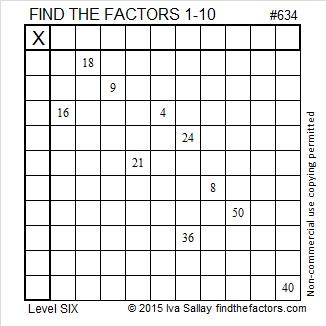# 634 and Level 6

634 is the sum of the eighteen prime numbers from 5 to 71. Can you name them all?

Every natural number greater than 2 is part of at least one Pythagorean triple. Where can we find 634 in a Pythagorean triple?

• 634 is the hypotenuse of only one Pythagorean triple: 150-616-634.
• Also 634 is a leg in exactly one triple: 634-100488-100490.

The greatest common factor for either triple is 2.Print the puzzles or type the solution on this excel file: 10 Factors 2015-09-28

—————————————————————————————————

• 634 is a composite number.
• Prime factorization: 634 = 2 x 317
• The exponents in the prime factorization are 1 and 1. Adding one to each and multiplying we get (1 + 1)(1 + 1) = 2 x 2 = 4. Therefore 634 has exactly 4 factors.
• Factors of 634: 1, 2, 317, 634
• Factor pairs: 634 = 1 x 634 or 2 x 317
• 634 has no square factors that allow its square root to be simplified. √634 ≈ 25.1793566.—————————————————————————————————